# K-Nearest Neighbor(KNN) Algorithm for Machine Learning### Sumer Pasha

##### 4 May 2023 - -4 min read

K-Nearest Neighbor(KNN) Algorithm for Machine Learning

• K-Nearest Neighbour is one of the simplest Machine Learning algorithms based on Supervised Learning techniques.
• K-NN algorithm assumes the similarity between the new case/data and available cases and put the new case into the category that is most similar to the available categories.
• K-NN algorithm stores all the available data and classifies a new data point based on the similarity. This means when new data appears then it can be easily classified into a good suite category by using K- NN algorithm.
• K-NN algorithm can be used for Regression as well as for Classification but mostly it is used for the Classification problems.
• K-NN is a non-parametric algorithm, which means it does not make any assumption on underlying data.
• It is also called a lazy learner algorithm because it does not learn from the training set immediately instead it stores the dataset and at the time of classification, it performs an action on the dataset.
• KNN algorithm at the training phase just stores the dataset and when it gets new data, then it classifies that data into a category that is much similar to the new data.
• Example: Suppose, we have an image of a creature that looks similar to a cat and a dog, but we want to know whether it is a cat or a dog. So for this identification, we can use the KNN algorithm, as it works on a similarity measure. Our KNN model will find the similar features of the new data set to the cats and dog’s images and based on the most similar features it will put it in either the cat or dog category.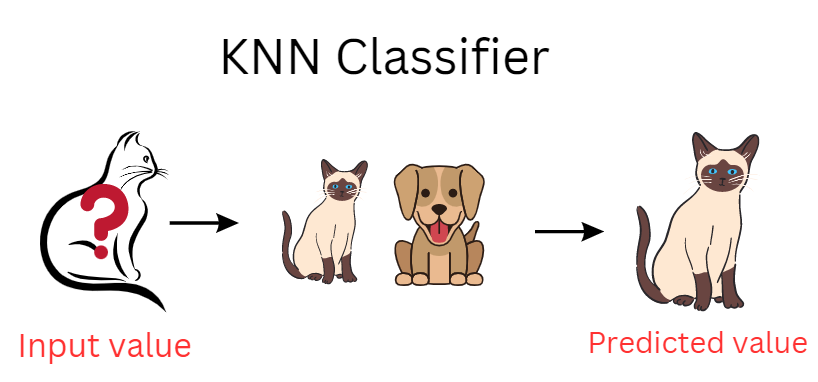Why do we need a K-NN Algorithm?

Suppose there are two categories, i.e., Category A and Category B, and we have a new data point x1, so this data point will lie in which of these categories? To solve this type of problem, we need a K-NN algorithm. With the help of K-NN, we can easily identify the category or class of a particular dataset. Consider the below diagram: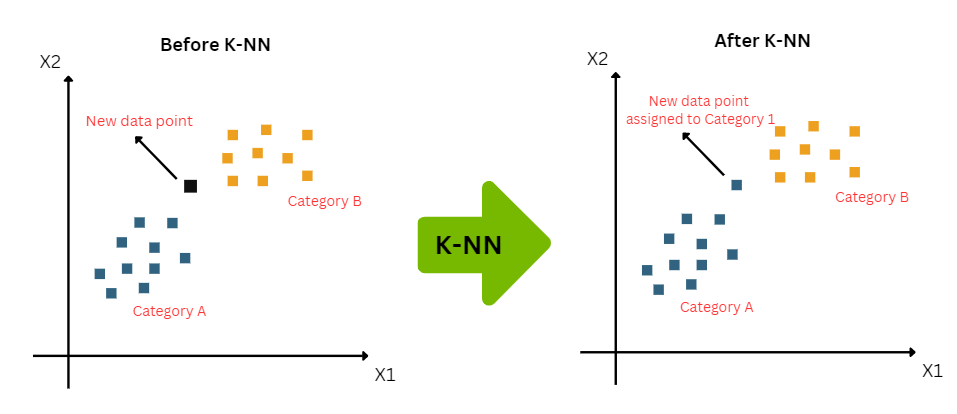How does K-NN work?

The K-NN working can be explained on the basis of the below algorithm:

• Step-1: Select the number K of the neighbors
• Step-2: Calculate the Euclidean distance of K number of neighbors
• Step-3: Take the K nearest neighbors as per the calculated Euclidean distance.
• Step-4: Among these k neighbors, count the number of the data points in each category.
• Step-5: Assign the new data points to that category for which the number of neighbors is maximum.
• Step-6: Our model is ready.

Suppose we have a new data point and we need to put it in the required category. Consider the below image: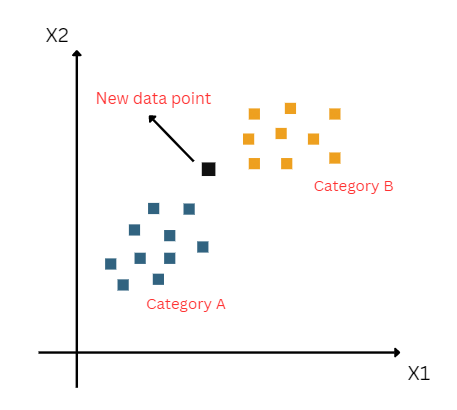• First, we will choose the number of neighbors so that we will choose the k=5.
• Next, we will calculate the Euclidean distance between the data points. The Euclidean distance is the distance between two points, which we have already studied in geometry. It can be calculated as: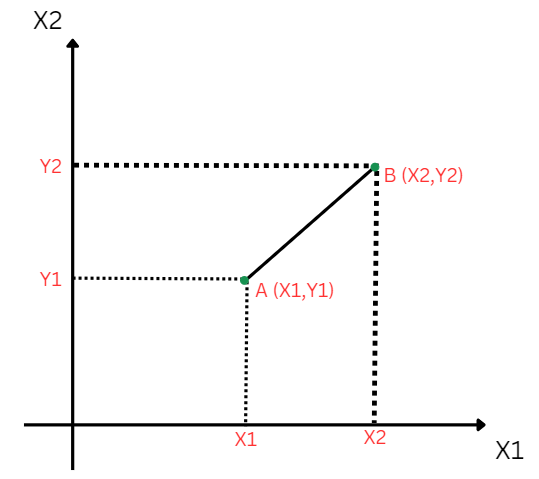• By calculating the Euclidean distance we got the nearest neighbors, as the three nearest neighbors in category A and the two nearest neighbors in category B. Consider the below image: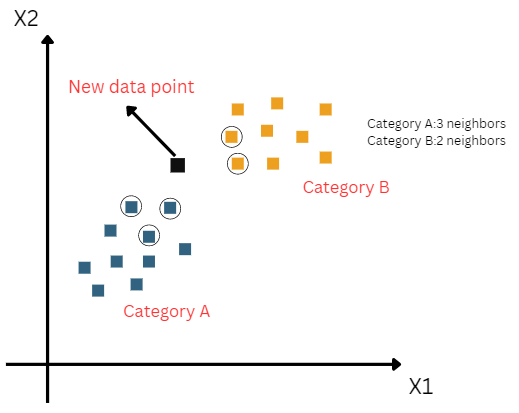• As we can see the 3 nearest neighbors are from category A, hence this new data point must belong to category A.

Why Value of K is Important ?

K affects the accuracy of our model and the number of nearest neighbors from which our new data is classified is highly significant very small value for K could lead to overfitting and a very small value for k could lead to underfitting. So, Choosing the right value for K is very important

How to select the value of K in the K-NN Algorithm?

Below are some points to remember while selecting the value of K in the K-NN algorithm:

• There is no particular way to determine the best value for " K", so we need to try some values to find the best out of them. The most preferred value for K is 5.
• A very low value for K such as K=1 or K=2, can be noisy and lead to the effects of outliers in the model.
• Large values for K are good, but it may find some difficulties.